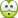# Tension of String

## Homework Statement

Hi, sorry, I am not good at writing English, but I am trying. I hope that someone helps me solve the following problem.

http://hodol.kr/q.jpg [Broken]

Please refer to the figure above.

A stick(AB) of length 5 is leaning against the Wall(AO) and a string(CO) is tied at the origin(O) and at a point(C) on the stick(AB). There are no frictional forces of wall and of floor so that the stick was sliding down. However, when the length of OB is 3, the sliding of the stick have been stoped. Figure out the strength of the tension force on the string(OC).

## The Attempt at a Solution

Well, I tried to solve the problem, but I could not. I think that the point C moves along some elliptic curve with origin at O. Therefore, the tension force on the string(OC) may be the same as the centripetal force of the movement along the elliptic curve and it makes balance with centrifugal force. Am I right, or please show me the solution.

Have a good day!
Thanks.

Last edited by a moderator:

billy_joule
Is that the problem statement word for word? It seems incomplete.
I would assume it's a static equilibrium problem. Apply the equations of static equilibrium and solve for T (in terms of m, the stick mass).
ΣFX =0
ΣFY =0
ΣM =0

stevendaryl
Staff Emeritus
You might be right, but I don't immediately see it.

The way I would do it is to use three equations and three unknowns: The unknowns are: (1) the force of the wall on the stick, (2) the force of the floor on the stick, and (3) the tension in the string. The equations are: (1) total force in the horizontal direction is zero. (2) total force in the vertical direction is zero. (3) torque on the stick is zero.

Chestermiller
Mentor
When they say "the sliding of the stick has been stopped," apparently what they mean is that it has been stopped manually, and not all by itself. So Billy Joule's and stevendaryl's interpretations would seem correct.

Chet

Thank you for kind replies, and I apologize for my bad English....

"the sliding of the stick has been stopped" does not mean that the stick stopped manually by external forces, but does mean that it stopped by the string(OC). You may think that the ends of the string are fixed at the point O and at the point C which divides the stick 4:1, and the string is an elastic band, and reached elastic limit at that moment, so that the elastic band will not become longer anymore.

Please, show me more details.stevendaryl
Staff Emeritus
Thank you for kind replies, and I apologize for my bad English....

"the sliding of the stick has been stopped" does not mean that the stick stopped manually by external forces, but does mean that it stopped by the string(OC). You may think that the ends of the string are fixed at the point O and at the point C which divides the stick 4:1, and the string is an elastic band, and reached elastic limit at that moment, so that the elastic band will not become longer anymore.

Please, show me more details.I think you need to use three variables: $T$ = tension in the string, $N_h$ = normal force of the wall on the stick, $N_v$ = normal force of the floor on the stick. Then you have three equations: (1) total force in vertical direction = 0, (2) total force in horizontal direction = 0, (3) torque (about whatever point you like) = 0.

So try to write down those three equations. (You'll also need $m$, the mass of the stick, and $g$, the acceleration of gravity)

•billy_joule and Chestermiller